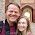Friday, October 30, 2015

The Traveling Vampire Problem

Let's say you are a vampire and you would like to figure out the shortest route to visit the supple

necks of N maidens. But, there is only so much time in any night!

You can fly from location to location, ignoring barriers.

With a few maidens, the problem is trivial.

However, as you entice more and more maidens you find the task of route management increasingly complex.

You buy a computer but find that using a blanket search algorithm to check all possible routes quickly becomes very time consuming as each additional maiden is added to the optimization.

The problem you realize is that each additional maiden increases the number of routes significantly. This is because there is a number of routes is equal to the permutation of N select N = N!.Four maidens, an easy problem.
So the number of routes:
1 maiden:   1=1
2 maidens: 1*2=2 (for example 1,2 or 2,1)
3 maidens: 1*2*3=6 (for example 1,2,3 or 1,3,2 or 2,1,3 or 2,3,1 or 3,2,1 or 3,1,2)
4 maidens: 1*2*3*4=24
5 maidens: 1*2*3*4*5=120
6 maidens: 1*2*3*4*5*6=720
7 maidens: 1*2*3*4*5*6*7=5,040
8 maidens: 1*2*3*4*5*6*7*8=40,320
9 maidens: 1*2*3*4*5*6*7*8*9=362,880
10 maidens:  1*2*3*4*5*6*7*8*9*10=3,628,800

As you start getting more and more maidens your algorithm to select the best route becomes extremely slow. You realize that using R your are going to face a practical limitation of spending as much time running the optimization as you will actually sucking necks. You know of Julia (which can run up to 500x faster than R) but you quickly realize that this is just postponing the problem. Even if you were running 500 times faster. Running the same algorithm on Julia is going to be four times faster after two more maidens (11*12/500=.26) but three times slower after 3 more maidens (11*12*13/500=3.4).Seven Maidens. Getting a bit more tricky.

You consider hibernating for a hundred years to see if computational speed increases will simplify the problem but also realize that if you keep approaching the problem using a binary computer with the same strategies as previously, you will always face similar computational limits. Eventually, and even very far into the future you will run out of computer speed long before you run out of maidens.

Being a clever vamp, you decide to start looking into alternative strategies to solving this kind of problem. But that is for another day.

--------------------------------

For what it is worth, I wrote a traveling vamp optimizer allowing for an arbitrary number dimensions to be specified. The most complex problem it solved was a 10 maiden problem and took a little over an hour.Two solutions for a 10 maiden problem. Top is shortest route while bottom is longest.
Find the code here.

1.Not certain if my first comment was actually posted. Why didn't you also employ R's TSP package - it contains a number of the standard TSP algorithms?

2.Your solution doesn't look optimal at the bottom left.

3.Im a lazy vampire that dont know enough statistics to improve this in any other way then speeding up the calculations.

So I changed your code a bit and got it to find the optimal routes to 10 maidens in 45 sec flat on my intel 3570K.

# Define a funtion to solve the traveling vamp problem
travelingvamp <- function(N,dim=2, visualize=FALSE) {
set.seed(1337)
# N is the number of maidens and dim is the dimensional space
# the default is 2 but you can imagine higher or lower dimensional as well

# Generate the matrix of maidens
maids <- matrix(rnorm(N*dim), ncol=dim)

# Write a function to minimize the euclidean distance.
# You could imagine alternative distance tools
euclid <- function (input){
input.clone <- input[-1,] - input[-nrow(input),]
sum <- apply(input.clone, 1, function(y) sqrt(sum(y^2)))
return (sum(sum))
}
# Calculate all of the alternative routes
routes <- permutations(N,N)

#routes <- mclapply(N, function (XX) permutations(XX,XX), mc.cores = getOption("mc.cores", 4L),mc.cleanup = TRUE, mc.allow.recursive = TRUE)
#routes <- matrix(unlist(routes), ncol=N)

# K is a measure of the number of routes
K <- prod(1:N)

# Create an empty vector of distance for each route
dist <- rep(NA, K)

# Calculate the distance needed to travel from the origin 0 to
# through the route
#for (i in 1:K) dist[i] <- euklides(rbind(0,maids[routes[i,],]))
#dist <- sapply(1:K, function (x) euklides(rbind(0,maids[routes[x,],])))
dist <- mclapply(1:K, function (x) euclid(rbind(0,maids[routes[x,],])), mc.cores = getOption("mc.cores", 4L), mc.cleanup = TRUE, mc.allow.recursive = TRUE)
dist <- unlist(dist)
# Find the minimum and the maximum distance routes
rmin <- routes[(dist==min(dist))]
rmax <- routes[(dist==max(dist))]

# If visualize is on, the graph both the quickest and longest routes
if (visualize) {
par(mfrow=c(2,1), mar=c(.5,0,0,0))
plot(rbind(0,maids[rmin,]), type='b', lwd=2, xaxt='n')
points(0,0, col="darkred", lwd=4)
par(mar=c(0,0,.5,0))
plot(rbind(0,maids[rmax,]), type='b', lwd=2, xaxt='n')
points(0,0, col="darkred", lwd=4)
mtext(paste("Round:",N, "#:", K, "Min:", round(min(dist),2),"Max:",round(max(dist),2)))
}

# Return the fastest and shortest routes
list(rmin=rbind(0,maids[rmin,]),
rmax=rbind(0,maids[rmax,])
)
}

1.Sweet, I will come back to this.

4.In the same vein.... http://www.slate.com/blogs/atlas_obscura/2015/12/02/using_math_to_calculate_how_long_it_would_take_vampires_to_annihilate_humanity.html